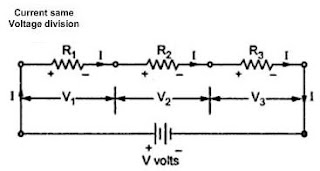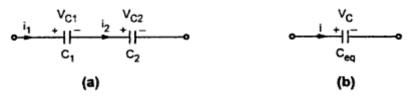### Series Circuit

A series circuit is one in which several resistances are connected one after the other. Such connection is also called end to end connection or cascade connection. There is only one path for the flow of current.
1. Resistor in series
Consider resistor shown in the Fig. 1. The resistance R1, R2 and R3 are said to be in series. The combination is connected across a source of a voltage V volts. Naturally the current flowing through all of them is same indicated as I amperes. e.g. the small chain of small lights, used for the decoration purposes is good example of series combination.Fig.  1  A series circuit
Now let us study the voltage distribution
Let V1, V2 and V3 be the voltages across the terminals of resistances R1, R2 and R3 respectively,
Then                                               V = V1 +V2 + V3
Now according to Ohm's law   V1 = I R1 ,     V2 = I R2     ,  V3 = I R3
Current through of them is same i.e. I
V = I R1 + I R2 + I R3 = I  (R1 +R2 +R3 )
Applying Ohm's law to overall circuit
V =  I Req
Where Req  = Equivalent resistance of the circuit, by comparison of tow equations,
Req = R+ R2 + R3
i.e. total or equivalent resistance of the series circuit is arithmetic sum of the resistances connected in series
For n resistances in series,                 R = R+ R2 + R3 +.......+ R
Characteristic of series circuits
1) The same current flows through each resistance.
2) The supply voltage V is the sum of the individual voltage drops across the resistances.
V = V1 +V2 + ..... + Vn
3) The equivalent resistance is equal to the sum of the individual resistances
4) The equivalent resistance is the largest of all the individual resistances.
i.e                                                  R >R ,  R >R2 , ..........R > Rn

2. Inductors in series
Consider in the Fig. 2(a). Tow inductors Land L2 are connected in series. The current flowing through L1 and L2 are i1 and i while voltages developed across L1 and L2 are VL1 and VL2  respectively. The equivalent circuit as shown in the Fig. 2(b).Fig.  2
For series combination,
i = i= i
and          V = VL1  + VL2
...              Leq  di/dt = L1 di/dt + L2 di/dt
...               Leq  di/dt = (L1 +L2 ) di/dt
...                Leq  = L1 +L2
That means, equivalent inductances of the series combination of tow inductances is the sum of inductances connected in series
The total equivalent inductances of the series circuit is the sum of the inductances connected in series.
For n inducatances in series,
Leq   = L1 + L2 + L3 +  ............+ Ln

3. Capacitors in series
Consider the Fig. 3(a). Tow capacitors C1 and C2 are connected in series. The current flowing through and voltages developed across C and C2 are i1, i2   and VC1  and VC2  respectively. The equivalent circuit is shown in the Fig. 3(b).Fig.  3

For series combination,
i = i1 = i2   and
V = VC1  + VC2
But                            i = i1 = i2

That means, reciprocal of equivalent capacitor of the series combination is the sum of  the reciprocal of individual capacitances.
The reciprocal of the total equivalent capacitors of the series combination is the sum of the reciprocols of the individual capacitors, connected in series,